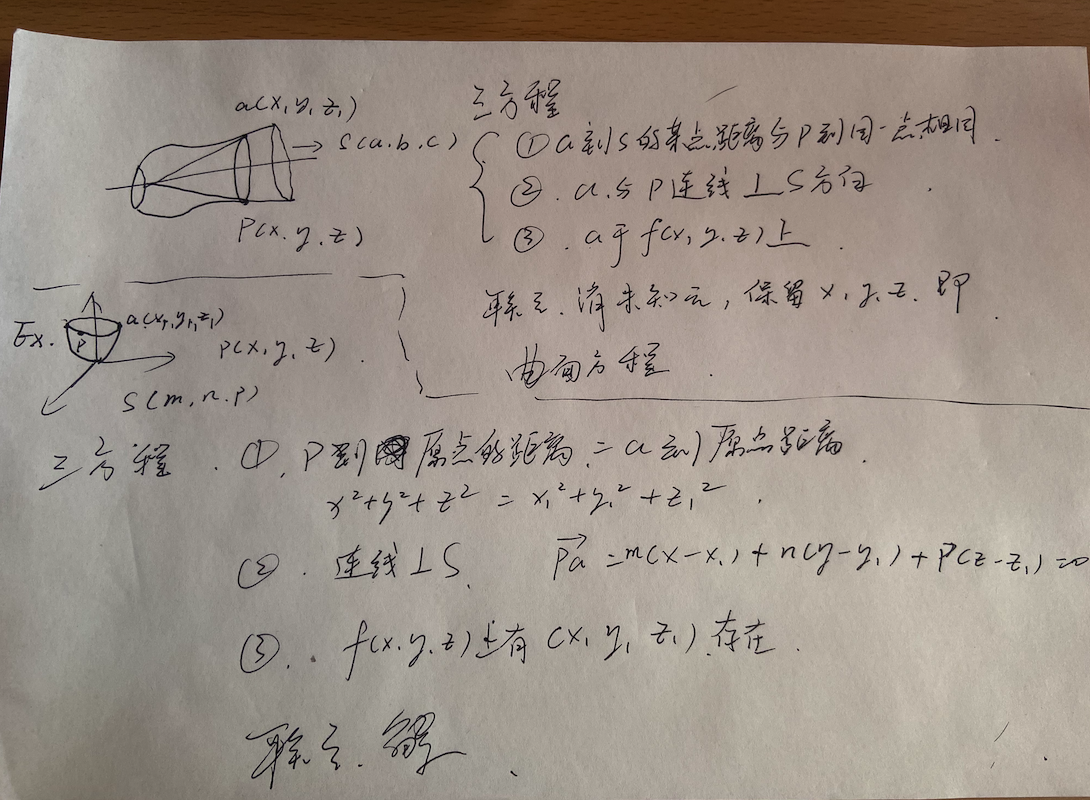# 曲线和空间

Author：张一极

20201202-11:16

## 曲面方程计算：

### 曲面方程计算流程（图丑）## 三重积分

### 直角坐标系和柱面坐标系的计算：

EX：

#### 柱面坐标系

$\begin{equation} \left\{\begin{array}{l} x=r \cos \theta \\ y=r \sin \theta \end{array}\right. \end{equation}$，如果在投影穿线法中的后部分$\begin{equation} \iint_{D_{n}} d \sigma \end{equation}$适用于极坐标系（被积函数为平方和，积分区域为圆）便有$\begin{equation} \iint_{a} f(x, y, z) \mathrm{d} x \mathrm{~d} y \mathrm{~d} z=\iint_{a} f(r \cos \theta, r \sin \theta, z) r \mathrm{~d} r \mathrm{~d} \theta \mathrm{d} z \end{equation}$

#### 球面坐标系1.换$dv=r^2sin\varphi dr$

2.换x,y,z：

3.逐层求定积分得到最后结果

## 第一型曲面积分

(1)一投：将 $\Sigma$ 投影到某一平面 $($ 比如 $x O y$$)$$\Rightarrow$ 投影区域为 $D\left(\right.$ 比如 $\left.D_{x y}\right)$找出投影区域的方式多种多样，一般是令某个区域的坐标为0，比如令z=0，得到xoy面的区域方程 ; (2)二代：将 $z=z(x, y)$ 或者 $F(x, y, z)=0$ 代人 $f(x, y, z)$; (3)三计算：计算z关于x和y的偏导数，带入弧面微分方程， $z_{x}^{\prime}, z_{y}^{\prime} \Rightarrow \mathrm{d} S=\sqrt{1+\left(z_{x}^{\prime}\right)^{2}+\left(z_{y}^{\prime}\right)^{2}} \mathrm{~d} x \mathrm{~d} y$ 这就把第一型曲面积分化成二重积分(如化成关于 $x, y$ 的二重积分 $)$,得到

## 曲线积分应用：

#### 重心，形心

$\bar{x}=\frac{\int_{L} x \rho(x, y, z) \mathrm{d} s}{\int_{L} \rho(x, y, z) \mathrm{d} s}, \quad \bar{y}=\frac{\int_{L} y \rho(x, y, z) \mathrm{d} s}{\int_{L} \rho(x, y, z) \mathrm{d} s}, \quad \bar{z}=\frac{\int_{L} z \rho(x, y, z) \mathrm{d} s}{\int_{L} \rho(x, y, z) \mathrm{d} s}$

$\bar{x}=\frac{\iint_{\Sigma} x \rho(x, y, z) \mathrm{d} S}{\iint_{\Sigma} \rho(x, y, z) \mathrm{d} S}, \quad \bar{y}=\frac{\iint_{\Sigma} y \rho(x, y, z) \mathrm{d} S}{\iint_{\Sigma} \rho(x, y, z) \mathrm{d} S}, \quad \bar{z}=\frac{\iint_{\Sigma} z \rho(x, y, z) \mathrm{d} S}{\iint_{\Sigma} \rho(x, y, z) \mathrm{d} S}$

$\bar{x}=\frac{\iiint_{\Omega} x \rho(x, y, z) \mathrm{d} v}{\iiint_{\Omega} \rho(x, y, z) \mathrm{d} v}，\bar{y}=\frac{\iiint_{\Omega} y \rho(x, y, z) \mathrm{d} v}{\iiint_{\Omega} \rho(x, y, z) \mathrm{d} v}，\bar{z}=\frac{\iiint_{\Omega} z \rho(x, y, z) \mathrm{d} v}{\iiint_{\Omega} \rho(x, y, z) \mathrm{d} v}$

$z=\frac{\iiint_{\Omega} z \mathrm{~d} v}{\iiint_{\Omega} d v}$

#### 转动惯量

$I_{x}=\int_{L}\left(y^{2}+z^{2}\right) \rho(x, y, z) \mathrm{d} s, \quad I_{y}=\int_{L}\left(z^{2}+x^{2}\right) \rho(x, y, z) \mathrm{d} s$ $I_{z}=\int_{L}\left(x^{2}+y^{2}\right) \rho(x, y, z) \mathrm{d} s, \quad I_{O}=\int_{L}\left(x^{2}+y^{2}+z^{2}\right) \rho(x, y, z) \mathrm{d} s$

$I_{x}=\iint_{D} y^{2} \rho(x, y) \mathrm{d} \sigma, \quad I_{y}=\iint_{D} x^{2} \rho(x, y) \mathrm{d} \sigma, \quad I_{O}=\iint_{D}\left(x^{2}+y^{2}\right) \rho(x, y) \mathrm{d} \sigma$

$I_{x}=\iiint_{\Omega}\left(y^{2}+z^{2}\right) \rho(x, y, z) \mathrm{d} v, \quad I_{y}=\iiint_{\Omega}\left(z^{2}+x^{2}\right) \rho(x, y, z) \mathrm{d} v$ $I_{z}=\iiint_{\Omega}\left(x^{2}+y^{2}\right) \rho(x, y, z) \mathrm{d} v, \quad I_{0}=\iiint_{\Omega}\left(x^{2}+y^{2}+z^{2}\right) \rho(x, y, z) \mathrm{d} v$

#### 引力密度

$F_{x}=G m \iint_{\Omega} \frac{\rho(x, y, z)\left(x-x_{0}\right)}{\left[\left(x-x_{0}\right)^{2}+\left(y-y_{0}\right)^{2}+\left(z-z_{0}\right)^{2}\right]^{\frac{3}{2}}} \mathrm{~d} v$

$F_{y}=G m \iiint_{\Omega} \frac{\rho(x, y, z)\left(y-y_{0}\right)}{\left[\left(x-x_{0}\right)^{2}+\left(y-y_{0}\right)^{2}+\left(z-z_{0}\right)^{2}\right]^{\frac{3}{2}}} \mathrm{~d} v$ $F_{z}=G m \iiint_{\Omega} \frac{\rho(x, y, z)\left(z-z_{0}\right)}{\left[\left(x-x_{0}\right)^{2}+\left(y-y_{0}\right)^{2}+\left(z-z_{0}\right)^{2}\right]^{\frac{3}{2}}} \mathrm{~d} v$

$\begin{array}{l} F_{x}=G m \int_{L} \frac{\rho(x, y, z)\left(x-x_{0}\right)}{\left[\left(x-x_{0}\right)^{2}+\left(y-y_{0}\right)^{2}+\left(z-z_{0}\right)^{2}\right]^{\frac{3}{2}}} \mathrm{~d} s \\ F_{y}=G m \int_{L} \frac{\rho(x, y, z)\left(y-y_{0}\right)}{\left[\left(x-x_{0}\right)^{2}+\left(y-y_{0}\right)^{2}+\left(z-z_{0}\right)^{2}\right]^{\frac{3}{2}}} \mathrm{~d} s \\ F_{z}=G m \int_{L} \frac{\rho(x, y, z)\left(z-z_{0}\right)}{\left[\left(x-x_{0}\right)^{2}+\left(y-y_{0}\right)^{2}+\left(z-z_{0}\right)^{2}\right]^{\frac{3}{2}}} \mathrm{~d} s \end{array}$

$\begin{array}{l} F_{x}=G m \iint_{\Sigma\left[\left(x-x_{0}\right)^{2}+\left(y-y_{0}\right)^{2}+\left(z-z_{0}\right)^{2}\right]^{\frac{3}{2}}} \mathrm{~d} S \\ F_{y}=G m \iint_{\Sigma\left[\left(x-x_{0}\right)^{2}+\left(y-y_{0}\right)^{2}+\left(z-z_{0}\right)^{2}\right]^{\frac{3}{2}}} \mathrm{~d} S \\ F_{z}=G m \iint_{\Sigma} \frac{\rho(x, y, z)\left(y-y_{0}\right)}{\left[\left(x-x_{0}\right)^{2}+\left(y-y_{0}\right)^{2}+\left(z-z_{0}\right)^{2}\right]^{\frac{3}{2}}} \mathrm{~d} S \end{array}$

## 第二型曲线积分：

### 计算

#### 直接转化为定积分

$\left\{\begin{array}{l}x=x(t) \\ y=y(t)\end{array}(t: \alpha \rightarrow \beta)\right.$，曲线方程如左，可以转换为：$\int_{L} P(x, y) \mathrm{d} x+Q(x, y) \mathrm{d} y=\int_{a}^{\beta}\left\{P[x(t), y(t)] \cdot x^{\prime} {(t)}+Q[x(t), y(t)] y^{\prime}(t)\right\} \mathrm{d} t$

## 第二型曲面积分

### 计算

#### 转化为二重积分

(1)将 $\Sigma$ 投影到某一平面(比如 $x O y$$)$$\Rightarrow$ 投影区域为 $D\left(\right.$ 比如 $\left.D_{x y}\right)$;

（2) 将 $z=z(x, y)$ 或者 $F(x, y, z)=0$ 代人 $R(x, y, z)$; （3) 将 $\mathrm{d} x \mathrm{~d} y$ 写成“ $\pm \mathrm{d} x \mathrm{~d} y$ ",其中 $\Sigma$ 方向向上(即法向量与 $z$ 轴夹角为锐角)时取“十”,否则取“ー"

#### 高斯公式

$\iiint_{\Omega}\left(\frac{\partial P}{\partial x}+\frac{\partial Q}{\partial y}+\frac{\partial R}{\partial z}\right) d v=\iint_{\Sigma} P d y d z+Q d z d x+R d x d y$ or

$\quad \iiint_{\Omega}\left(\frac{\partial P}{\partial x}+\frac{\partial Q}{\partial y}+\frac{\partial R}{\partial z}\right) d v$$\quad=\iint_{\Sigma}(P \cos \alpha+Q \cos \beta+R \cos \gamma) d S$

## 空间第二型曲线

### 斯托克斯公式

(1)曲面 $\Sigma$ 在点 $P_{0}\left(x_{0}, y_{0}, z_{0}\right)$ 处的法向量(垂直于该点切平面的向量)为

$\quad$$\Omega$ 为空间某区域, $\Sigma$$\Omega$ 内的分片光滑有向曲面片, $l$ 为逐段光滑的 $\Sigma$ 的边界,它的方向与 $\Sigma$ 的法向量成右手系,函数 $P(x, y, z), Q(x, y, z)$$R(x, y, z)$$\Omega$ 内具有连续的一阶偏导数,则 有斯托克斯公式:

$\oint_{I} P \mathrm{~d} x+Q \mathrm{~d} y+R \mathrm{~d} z=\iint_{\Sigma}\left|\begin{array}{ccc}\cos \alpha & \cos \beta & \cos \gamma \\ \frac{\partial}{\partial x} & \frac{\partial}{\partial y} & \frac{\partial}{\partial z} \\ P & Q & R\end{array}\right| \mathrm{d} S$

## 问题集合：

1. $\Sigma$ 为球面 $x^{2}+y^{2}+z^{2}=1,$ 则第一型曲面积分 $\iint_{\Sigma} x(4 x-z) \mathrm{d} S=$

2. $\Sigma$ 是球面 $x^{2}+y^{2}+z^{2}=a^{2}(a>0)$ 的外侧, 则 $\oiint_{\Sigma} x y^{2} \mathrm{~d} y \mathrm{~d} z+y z^{2} \mathrm{~d} z \mathrm{~d} x+z x^{2} \mathrm{~d} x \mathrm{~d} y=$

(本题不可以将积分区域范围直接代入被积函数，因为在空间内部，$x^2+y^2+z^2$未必一定等于$a^2$，只有他们在整个区域上相同的时候才可以直接换元)